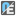• # How is TZ decrypted?

## Question related to missionBit Message

I have succeed in solving the problem except for getting the correct time zone. I have no problem for getting the + or - sign, but not the timezone number.

+7 = 28 quarters, so 28 in binary should be 11100, but 82 in the code translate to 1000 0010.

+4 and -4 = 16 quarters (10000), but the code is 0110 1001 and 0110 0001.

-11 = 44 quarters (101100), the code is 0100 1100.

I dont see the pattern here. Could some one explain please?